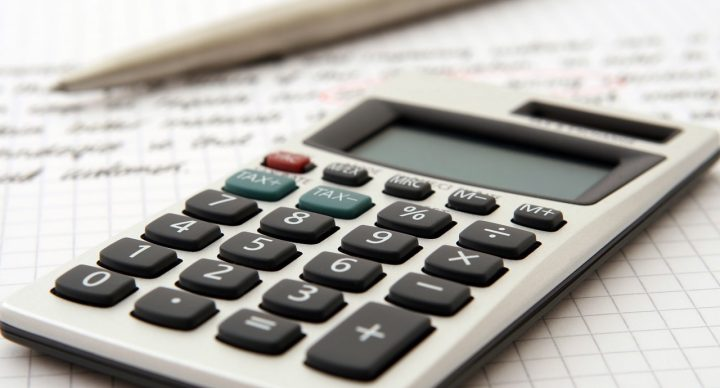# AP Calculus AB Exam Prep### Course Overview

The AP Calculus AB Exam Prep course was designed for students to quickly and efficiently review many concepts typically covered on the AP Calculus AB Exam. After being shown step-by-step how to solve several carefully selected exam-like problems on a particular topic, students are given similar problems to cement their understanding. Two videos are provided for each lesson, giving students a "fast track" option as well as additional, more detailed instruction. The AP Calculus AB Exam Prep course:
• Consists of short, concise lessons
• Presents problems modeled after those given in previous AP Calculus AB Exams
• Provides may different types of problems, some requiring an analytical (algebraic) approach, others requiring numerical analysis or a graphical solution
• Covers both multiple choice and free-response types of problems
• Incorporates both calculator active and non-calculator active types of problems
• Provides helpful strategies to be used when taking the AP Calculus AB Exam
• Includes information on exam grading
• Contains suggestions on how to make final preparations prior to taking the exam
• Gives students extra confidence going into the exam
The AP Calculus AB Exam Prep course was developed by Patrick Mara, who is the instructor for the Acellus AP Calculus course. (The Acellus AP Calculus course is authorized by the College Board for the use of "AP" in the title.) Patrick Mara is a veteran AP Calculus teacher, and has been an AP exam reader in past years. He has an excellent background as to the key topics and types of questions that are covered on the exam. This course can be used either in conjunction with Acellus AP Calculus or as a stand-alone preparation course following other AP Calculus AB curriculums.

### Scope and Sequence

This course presents a comprehensive review of AP Calculus AB, including a discussion about taking the exam, as well as derivatives, equation of tangent line, implicit differential, antidifferentiation, limits, 1st derivative analysis, f' and f" analysis, continuity and differentiability. Also covered are related rates, fundamental theorems of calculus, position, velocity, acceleration, and speed, as well as inverse functions, chain rule, average value, definite integrals, volumes of solids, differential equations, approximating area, mean, extreme, and intermediate value theorem, multiple representations of functions, data presented in tables, implicit differentiation, and slope fields.This course was developed by the International Academy of Science. Learn More